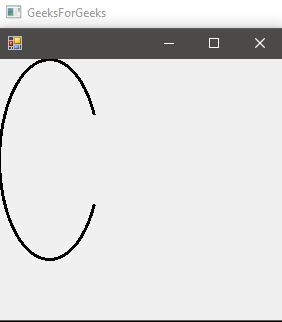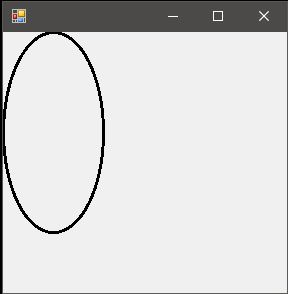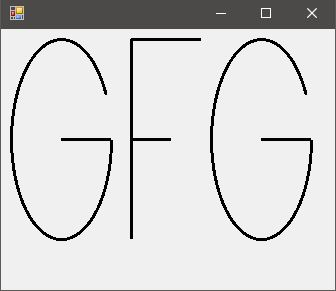Related Articles

# Graphics.DrawArc() Method in C# with Examples

• Last Updated : 04 Apr, 2019

Graphics.DrawArc Method is used to draw an arc representing a portion of an ellipse specified by a pair of coordinates, a width, and a height. There are 4 methods in the overload list of this method as follows:

• DrawArc(Pen, Rectangle, Single, Single) Method
• DrawArc(Pen, RectangleF, Single, Single) Method
• DrawArc(Pen, Int32, Int32, Int32, Int32, Int32, Int32) Method
• DrawArc(Pen, Single, Single, Single, Single, Single, Single) Method

#### DrawArc(Pen, Rectangle, Single, Single)

This method is used to draw an arc representing a portion of an ellipse specified by a Rectangle structure.

Syntax: public void DrawArc (System.Drawing.Pen pen, System.Drawing.Rectangle rect, float startAngle, float sweepAngle)

Parameters:
pen: Determines the color, width, and style of the arc.
rect: Determines the smallest rectangle in which the ellipse just perfectly fits into.
startAngle: Angle in degrees measured clockwise from the x-axis to the starting point of the arc.
sweepAngle: Angle in degrees measured clockwise from the startAngle parameter to ending point of the arc.

Exception: This method will give ArgumentNullException if the pen is null.

Example:

 `// C# program to illustrate the ``// DrawArc(Pen, Rectangle, Single,``// Single) Method``using` `System;``using` `System.Drawing;``using` `System.Drawing.Printing;``using` `System.Windows.Forms;`` ` `namespace` `GFG {`` ` `class` `PrintableForm : Form {`` ` `    ``// Main Method``    ``public` `static` `void` `Main()``    ``{``        ``Application.Run(``new` `PrintableForm());``    ``}``    ``public` `PrintableForm()``    ``{``        ``ResizeRedraw = ``true``;``    ``}`` ` `    ``protected` `override` `void` `OnPaint(PaintEventArgs e)``    ``{``        ``// Create pen.``        ``Pen blackPen = ``new` `Pen(Color.Black, 3);`` ` `        ``// Reactangle with specifies x1,``        ``// y1, x2, y2 respectively``        ``Rectangle rect = ``new` `Rectangle(0, 0, 100, 200);`` ` `        ``// Create start and sweep angles on ellipse.``        ``float` `startAngle = 45.0F;``        ``float` `sweepAngle = 270.0F;`` ` `        ``// Draw arc to screen.``        ``e.Graphics.DrawArc(blackPen, rect, ``                  ``startAngle, sweepAngle);``    ``}``}``}`

Output:#### DrawArc(Pen, RectangleF, Single, Single)

This method is used to draw an arc representing a portion of an ellipse specified by a RectangleF structure.

Syntax: public void DrawArc (System.Drawing.Pen pen, System.Drawing.RectangleF rect, float startAngle, float sweepAngle);

Parameters:
pen: Determines the color, width, and style of the arc.
rect: Determines the smallest rectangle in which the ellipse just perfectly fits into.
startAngle: Angle in degrees measured clockwise from the x-axis to the starting point of the arc.sweepAngle: Angle in degrees measured clockwise from the startAngle parameter to ending point of the arc.

Exception: This method will give ArgumentNullException if the pen is null.

Example 1:

 `// C# program to illustrate the ``// DrawArc(Pen, RectangleF, ``// Single, Single) Method``using` `System;``using` `System.Drawing;``using` `System.Drawing.Printing;``using` `System.Windows.Forms;`` ` `namespace` `GFG {`` ` `class` `PrintableForm : Form {`` ` `    ``// Main Method``    ``public` `static` `void` `Main()``    ``{`` ` `        ``Application.Run(``new` `PrintableForm());``    ``}`` ` `    ``public` `PrintableForm()``    ``{``        ``ResizeRedraw = ``true``;``    ``}`` ` `    ``protected` `override` `void` `OnPaint(PaintEventArgs e)``    ``{``        ``// Create pen``        ``Pen blackPen = ``new` `Pen(Color.Black, 3);`` ` `        ``// Reactangle with specifies x1,``        ``// y1, x2, y2 respectively``        ``RectangleF rect = ``new` `RectangleF(0.0F, ``                        ``0.0F, 100.0F, 200.0F);`` ` `        ``// Create start and sweep``        ``// angles on an ellipse.``        ``float` `startAngle = 45.0F;``        ``float` `sweepAngle = 270.0F;`` ` `        ``// Draw arc to screen.``        ``e.Graphics.DrawArc(blackPen, rect,``                  ``startAngle, sweepAngle);``    ``}``}``}`

Output:#### DrawArc(Pen, Single, Single, Single, Single, Single, Single)

This method is used to draw an arc representing a portion of an ellipse specified by a pair of coordinates, a width, and a height.

Syntax: public void DrawArc (System.Drawing.Pen pen, float x, float y, float width, float height, float startAngle, float sweepAngle);

Parameters:
pen: Pen determines the color, width, and style of the line.
x: The abscissa of the upper-left corner of the rectangle that defines the ellipse.
y: The ordinate of the upper-left corner of the rectangle that defines the ellipse.
width: Width of the rectangle that defines the ellipse or more specifically we can say the diameter of the ellipse in abscissa.
height: Height of the rectangle that defines the ellipse or more specifically we can say the diameter of the ellipse in ordinate.
startAngle: Angle in degrees measured clockwise from the x-axis to the starting point of the arc.
sweepAngle: Angle in degrees measured clockwise from the startAngle parameter to the ending point of the arc.

Exception: This method will give ArgumentNullException if the pen is null.

Example 1:

 `// C# program to draw a circle``using` `System;``using` `System.Drawing;``using` `System.Drawing.Printing;``using` `System.Windows.Forms;`` ` `namespace` `GFG {`` ` `class` `PrintableForm : Form {`` ` `    ``// Main Method``    ``public` `static` `void` `Main()``    ``{``        ``Application.Run(``new` `PrintableForm());``    ``}`` ` `    ``public` `PrintableForm()``    ``{``        ``ResizeRedraw = ``true``;``    ``}`` ` `    ``protected` `override` `void` `OnPaint(PaintEventArgs e)``    ``{``        ``// Create pen.``        ``Pen blackPen = ``new` `Pen(Color.Black, 3);`` ` `        ``// Create coordinates of the rectangle``        ``// to the bound ellipse.``        ``int` `x = 0;``        ``int` `y = 0;``        ``int` `width = 100;``        ``int` `height = 200;`` ` `        ``// Create start and sweep ``        ``// angles on ellipse.``        ``int` `startAngle = 0;``        ``int` `sweepAngle = 360;`` ` `        ``// Draw arc to screen.``        ``e.Graphics.DrawArc(blackPen, x, y, width,``                 ``height, startAngle, sweepAngle);``    ``}``}``}`

Output:Example 2:

 `// C# program to draw GFG``using` `System;``using` `System.Drawing;``using` `System.Drawing.Printing;``using` `System.Windows.Forms;`` ` `namespace` `GFG {`` ` `class` `PrintableForm : Form {`` ` `    ``// Main Method``    ``public` `static` `void` `Main()``    ``{``        ``Application.Run(``new` `PrintableForm());``    ``}`` ` `    ``public` `PrintableForm()``    ``{``        ``ResizeRedraw = ``true``;``    ``}`` ` `    ``protected` `override` `void` `OnPaint(PaintEventArgs e)``    ``{``        ``// Create pen.``        ``Pen blackPen = ``new` `Pen(Color.Black, 3);`` ` `        ``// Creates letter G``        ``e.Graphics.DrawArc(blackPen, 10,``                  ``10, 100, 200, 0, 315);`` ` `        ``e.Graphics.DrawLine(blackPen,``                  ``60, 110, 110, 110);`` ` `        ``// Creates letter F``        ``e.Graphics.DrawLine(blackPen,``                  ``130, 10, 130, 210);`` ` `        ``e.Graphics.DrawLine(blackPen,``                   ``130, 10, 200, 10);`` ` `        ``e.Graphics.DrawLine(blackPen, ``                 ``130, 110, 170, 110);`` ` `        ``// Creates the next letter G``        ``e.Graphics.DrawArc(blackPen,``         ``210, 10, 100, 200, 0, 315);`` ` `        ``e.Graphics.DrawLine(blackPen,``                 ``260, 110, 310, 110);``    ``}``}``}`

Output:#### DrawArc(Pen, Int32, Int32, Int32, Int32, Int32, Int32)

This method is used to draw an arc representing a portion of an ellipse specified by a pair of coordinates, a width, and a height.

Syntax: public void DrawArc (System.Drawing.Pen pen, int x, int y, int width, int height, int startAngle, int sweepAngle);

Parameters:
pen: Pen determines the color, width, and style of the line.
x: The abscissa of the upper-left corner of the rectangle that defines the ellipse.
y: The ordinate of the upper-left corner of the rectangle that defines the ellipse.
width: Width of the rectangle that defines the ellipse or more specifically we can say the diameter of the ellipse in abscissa.
height: Height of the rectangle that defines the ellipse or more specifically we can say the diameter of the ellipse inordinate.
startAngle: Angle in degrees measured clockwise from the x-axis to the starting point of the arc.
sweepAngle: Angle in degrees measured clockwise from the startAngle parameter to ending point of the arc.

Exception: This method will give ArgumentNullException if the pen is null.

Example:

 `// C# program to draw a circle``using` `System;``using` `System.Drawing;``using` `System.Drawing.Printing;``using` `System.Windows.Forms;`` ` `namespace` `GFG {`` ` `class` `PrintableForm : Form {`` ` `    ``// Main Method``    ``public` `static` `void` `Main()``    ``{``        ``Application.Run(``new` `PrintableForm());``    ``}``    ``public` `PrintableForm()``    ``{``        ``ResizeRedraw = ``true``;``    ``}``    ``protected` `override` `void` `OnPaint(PaintEventArgs e)``    ``{``        ``// Create pen.``        ``Pen blackPen = ``new` `Pen(Color.Black, 3);`` ` `        ``// Create coordinates of the rectangle``        ``// to the bound ellipse.``        ``float` `x = 0.0F;``        ``float` `y = 0.0F;``        ``float` `width = 100.0F;``        ``float` `height = 200.0F;`` ` `        ``// Create start and sweep``        ``// angles on the ellipse.``        ``float` `startAngle = 0.0F;``        ``float` `sweepAngle = 360.0F;`` ` `        ``// Draw arc to screen.``        ``e.Graphics.DrawArc(blackPen, x, y, width,``                 ``height, startAngle, sweepAngle);``    ``}``}``}`

Output:Reference:

My Personal Notes arrow_drop_up